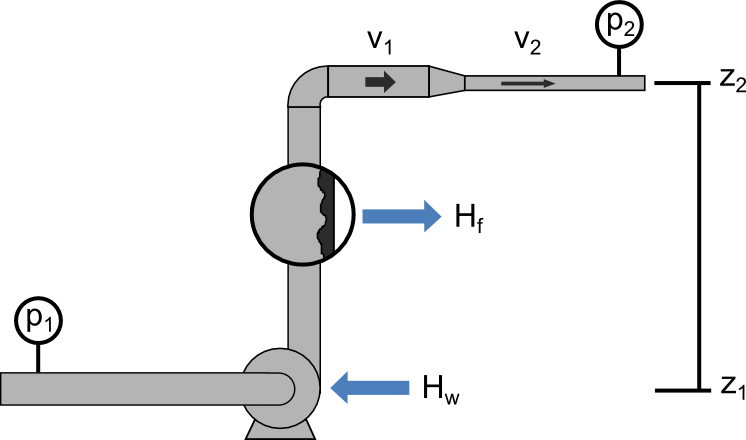# Summary

Bernoulli’s Principle is an important observation in fluid dynamics which states that for an inviscid flow, an increase in the velocity of the fluid results in a simultaneous decrease in pressure or a decrease in the fluid’s potential energy. This principle is often represented mathematically in the many forms of Bernoulli’s equation. This article presents some useful forms of Bernoulli’s Equations and their simplifying assumptions.

# Introduction

Bernoulli’s Principle is an application of the first law of thermodynamics, the conservation of energy, to fluid systems. It describes the conversion of energy within a system in terms of pressure, velocity, and height.

Expanded forms of this equation may additionally account for energy lost or gained as work or heat and mass accumulated in the system. There are many forms in which Bernoulli’s principle may be written. Several common forms of Bernoulli’s equation are shown below.# Useful Forms of the Bernoulli Equation

## Incompressible Flow Expressed in Fluid Head Units

For liquid systems it is often safe to assume the fluid is incompressible and that the system is at steady state. For the study of liquid systems a common unit of measure is fluid head, which represents all forms of energy as the equivalent height to which the fluid must be raised to have an equivalent potential energy due to gravity.

### Assumptions

• Incompressible fluid
• Single inlet
• Single outlet
• Measured in units of head (length, e.g. meters)

### Equation Forms

Based on these assumptions the energy balance equation in terms of fluid head is:

Where,

Substituting the above we have a common form of the equation used when dealing with incompressible fluid systems with single inlets and outlets:

## Steady-state Single Inlet and Outlet Expressed in Energy Units

For a single inlet and outlet systems at steady state, the equation may be simplified and expressed in terms of absolute energy. When working with compressible fluids units of energy must be used as fluid head is not appropriate in situations where density is not constant. Working in units of energy is also suitable for incompressible fluids.

### Assumptions

• Compressible or incompressible fluid
• Single inlet
• Single outlet
• Measured in units of energy (e.g. or )

### Equation Forms

In units of specific energy ( ):

In units of energy ( ):

## Energy Conservation for Any System

The energy balance can be generalised for systems with multiple inputs and outputs as well as changes in mass and energy of the system (accumulation or depletion).

### Assumptions

• Compressible or incompressible fluid
• Accumulation or depletion of system mass or energy
• Multiple inlet streams
• Multiple outlet streams

### Equation Form

For any system the energy balance may be expressed as follows:

1. Perry's Chemical Engineers' Handbook, Eighth Edition2. Chemical Engineering Volume 1, Sixth Edition: Fluid Flow, Heat Transfer and Mass Transfer (Coulson & Richardson's Chemical Engineering)3. Fluid Flow for Chemical and Process Engineers, Second Edition